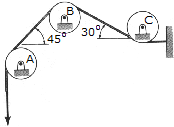# Engineering Mechanics - Equilibrium of a Rigid Body - Discussion

### Discussion :: Equilibrium of a Rigid Body - General Questions (Q.No.12)

12.Compute the horizontal and vertical components of force at pin B. The belt is subjected to a tension of T=100 N and passes over each of the three pulleys.

 [A]. Bx = 0 N, By = 141.4 N [B]. Bx = -15.89 N, By = 120.7 N [C]. Bx = 20.7 N, By = 157.3 N [D]. Bx = 0, By = 100.0 N

Explanation:

No answer description available for this question.

 Mayuresh Date said: (Jul 23, 2013) Consider equilibrium of pulley B(joint B), the tension of 100N is acting on both sides at an angle 45 deg and 30 deg,apply laws of statics as follows. -T.sin45 - T.sin30 + By = 0. & T.cos30 - Tcos45 + Bx = 0. Where T = 100N.